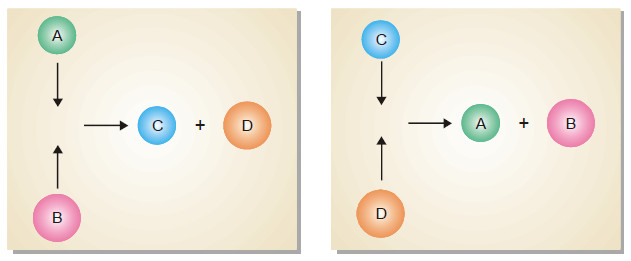# Characteristics of Chemical Equilibrium

Chemical Equilibrium is the state of a reversible reaction when the two opposing reactions occur at the same rate and the concentrations of reactants and products do not change with time.

## Nature of Chemical Equilibrium : its definition

– Let us consider the reaction

A + B ↔ C + D

– If we start with A and B in a closed vessel, the forward reaction proceeds to form C and D.

– The concentrations of A and B decrease and those of C and D increase continuously.

– As a result the rate of forward reaction also decreases and the rate of the reverse reaction increases.

– Eventually, the rate of the two opposing reactions equals and the system attains a state of equilibrium.

– Thus Chemical equilibrium may be defined as: the state of a reversible reaction when the two opposing reactions occur at the same rate and the concentrations of reactants and products do not change with time.

– Furthermore, the true equilibrium of a reaction can be attained from both sides.

– Thus the equilibrium concentrations of the reactants and products are the same whether we start with A and B, or C and D.

## Chemical Equilibrium is Dynamic Equilibrium

– We have shown above that as the reaction A + B ↔ C + D attains equilibrium, the concentrations of A and B, as also of C and D remain constant with time.

– Apparently it appears that the equilibrium is dead. But it is not so. The equilibrium is dynamic.

– Actually, the forward and the reverse reactions are taking place at equilibrium but the concentrations remain unchangedMolecules of A and B colliding to give C and D, and those of C and D colliding to give A and B.

– The dynamic nature of chemical equilibrium can be easily understood on the basis of the kinetic molecular model.

– The molecules of A and B in the equilibrium mixture collide with each other to form C and D. Likewise C and D collide to give back A and B.

– The collisions of molecules in a closed system is a ceaseless phenomenon. Therefore collisions of A and B giving C and D (Forward reaction) and collisions of C and D giving back A and B (reverse reaction) continue to occur even at equilibrium, while concentrations remain unchanged.

## Characteristics of Chemical Equilibrium

– Before we take up the mathematical study of chemical equilibria, let us understand the chemical characteristics of chemical equilibrium.

### (1) Constancy of concentrations

– When a chemical equilibrium is established in a closed vessel at constant temperature, concentrations of the various species in the reaction mixture become constant.

– The reaction mixture at equilibrium is called Equilibrium mixture.

– And The concentrations at equilibrium are called Equilibrium concentrations.

– The equilibrium concentrations are represented by square brackets with subscript eq or eqm, [ ]eq.

– Thus [A]eq denotes the equilibrium concentration of substance A in moles per litre.

– In modern practice the subscript eq is not used.

### (2) Equilibrium can be initiated from either side

– The state of equilibrium of a reversible reaction can be approached whether we start with reactants or products, for example, the equilibrium:

H2 (g) + I2(g) ↔ 2HI(g)

is established if we start the reaction with H2 and I2, or 2HI.

– The following figure Shows whether we start with 1 mole of I2 and 1 mole of H2, or with 2 moles of HI in a vessel of the same volume, the same mixture of H2, I2 and HI is obtained.

### (3) Equilibrium cannot be Attained in an Open Vessel

– The equilibrium can be established only if the reaction vessel is closed and no part of the reactants or products is allowed to escape out.

– In an open vessel, the gaseous reactants and/or products may escape into the atmosphere leaving behind no possibility of attaining equilibrium. However, the equilibrium can be attained when all the reactants and products are in the same phase i.e., ethanol and ethanoic acid.

### (4) A catalyst cannot change the equilibrium point

– When a catalyst is added to a system in equilibrium, it speeds up the rate of both the forward and the reverse reaction to an equal extent.

– Therefore a catalyst cannot change the equilibrium point except that it is achieved earlier. This enhances the rate of the reaction.

– The rapid increase of the rate of an equilibrium reaction on the addition of a catalyst is illustrated in the following figure: